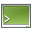# How to Estimate Memory Requirements for the MLFMM

A method is presented that allows you to estimate the memory requirements for a model solved using the multilevel fast multipole method (MLFMM).

The MLFMM memory requirement is directly proportional to $N{\mathrm{log}}_{10}\left(N\right)$ , where $N$ is the number of unknowns. When the frequency doubles, the triangle size in the mesh should be halved (to keep the same triangle size in terms of the wavelength). This causes the number of unknowns to increase by a factor of 4.

For example, if a model has 10 000 unknowns at 200 MHz, it will have 40 000 unknowns after meshing for 400 MHz. The increase in memory for the MLFMM solver is then:

(1) $\frac{40000×{\mathrm{log}}_{10}\left(40000\right)}{10000×{\mathrm{log}}_{10}\left(10000\right)}=4.8$

For models with increasing electrical size, the above equation becomes less accurate. In addition to the number of unknowns, the total memory requirement also depends on the following:
• Uniformity of the mesh (similar element sizes for the whole mesh versus a mixture containing very fine element sizes)
• Number of mesh elements, specifically the storage of the triangle information (data such as position, normal, size)
• Number of parallel processes
• Number of nodes (hosts) used in a compute cluster scenario1
• Type and size of the preconditioner
Note: This how-to was created using Feko version 2019 that includes several shared memory upgrades for parallel processing. Always ensure that you are using the latest Feko version.

## Steps to Estimate the Memory

An optional parameter for the solution method allows you to calculate an estimate for the required memory.

1. On the Solve/Run tab, in the Run/Launch group, click theFeko Terminal icon.
2. Run the Solver using the intended number of processes using the special execution mode:
--estimate-resource-requirements-only
3. When the Solver execution is complete, open the .out file and find the following text block at the end of the file:
Peak memory usage during the whole solution: 61.707 MByte
(refers to the master process only)
Sum of the peak memory of all processes:     1.928 GByte
On average per process:                      61.698 MByte

NOTE    48414: Memory requirement for a regular Feko run (without --estimate-resource-requirements-only) is higher

Memory estimate for a regular Feko run:      59.784 GByte (total for all parallel processes)
On average per process:                      1.868 GByte
4. The estimated memory is given by Memory estimate for a regular Feko run.

## Example

Solve a model with the file name car_a.cfx using 40 processes on a cluster with two hosts. Each host has a total of 20 available cores. The machines file name is hosts.list.

Open a Feko Terminal window on the host (or master node on the cluster) and execute the following command:
runfeko car_a -np 40 --machines-file hosts.list --feko-options --estimate-resource-requirements-only

## Notes on Usage

• If the model is to be solved on a cluster, the correct machines file should be used as some aspects of the solution are duplicated on each node.

If the estimation algorithm is executed with the intended number of parallel processes, but only on a single node, the estimate is less accurate compared to execution over all the intended nodes.

• When comparing the execution time for the estimation algorithm to the solution of the model, the estimation algorithm is only a fraction of the total run time.
• The estimation algorithm requires roughly between 1/30th and 1/3rd of the memory for a complete solution.2
• If a memory error occurs during the estimate, the full solution will also fail on the same host / cluster that the estimation was performed with, for example:
ERROR   32463: Not enough memory available for dynamic allocation
• An increase in the number of processes results in a less accurate estimate as some solution aspects are duplicated for each parallel process, but not included in the estimate.
Note: For example, solving a very large model comprising several tens of millions of triangles with:
• 32 processes, the estimate was 90% accurate
• 256 processes, the estimate was 85% accurate
• The estimation is available for both SPAI and sparse LU preconditioners for sequential and parallel solutions.
• Output requests (for example, far field requests) increases the memory requirement and are not included in the estimation.
1 Some aspects of the solution are copied to each process and / or to each node.
2 Based on a few limited comparisons.